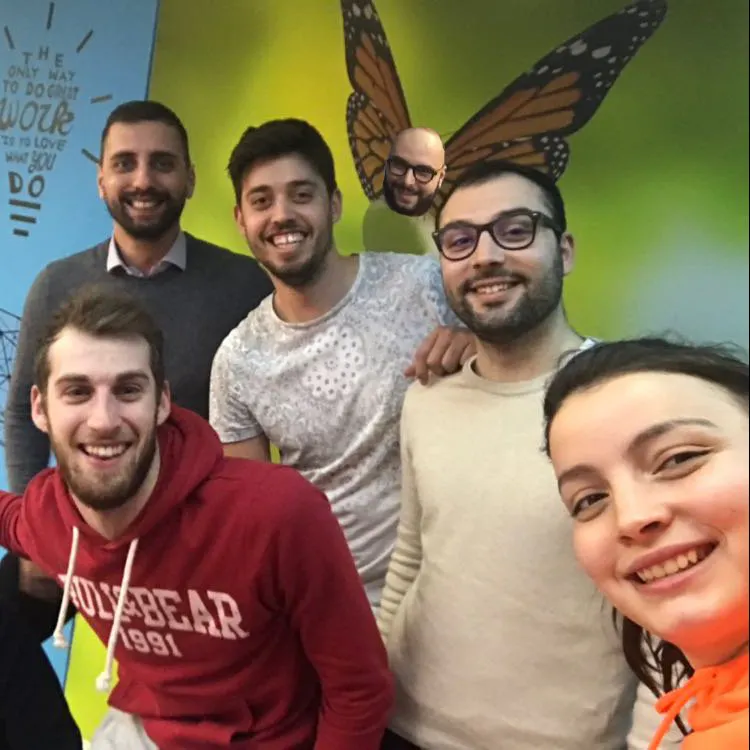# Alexa Humour DJ

Chooses the best song and the best illumination type based on your current mood.

IntermediateFull instructions provided5 hours2,129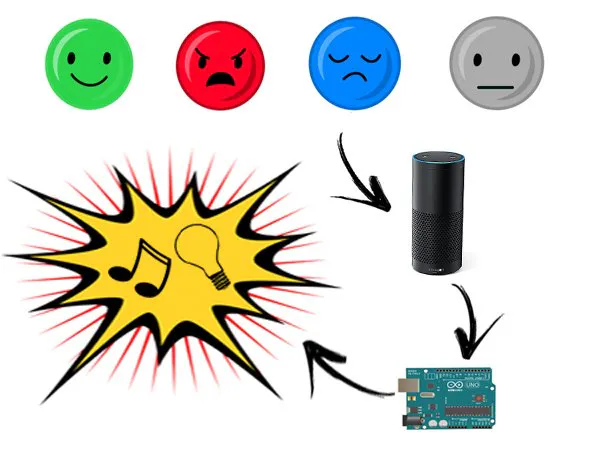## Things used in this project

### Hardware componentsRaspberry Pi 3 Model B
×1Arduino UNO & Genuino UNO
×1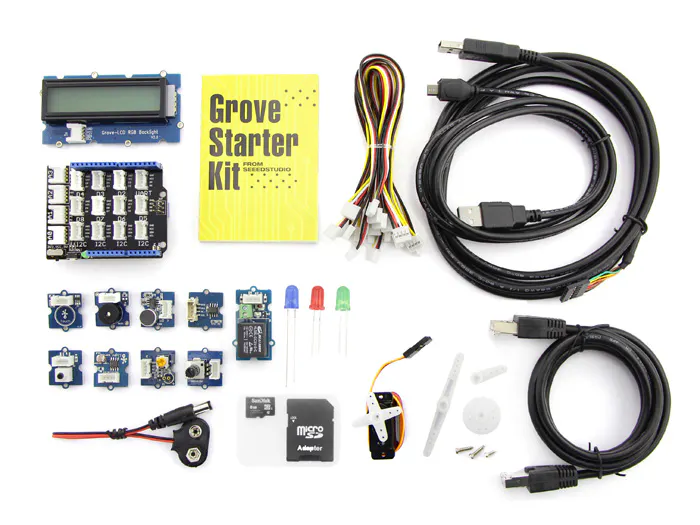Seeed Grove starter kit plus for Intel Edison
×1×1LED (generic)
×8
 Seeed RGB Led
×1
 Seeed Sound sensor
×1

### Software apps and online servicesAmazon Alexa Alexa Skills KitAmazon Alexa Alexa Voice Service

### Hand tools and fabrication machines3D Printer (generic)

## Schematics

### HW Schema

Project schema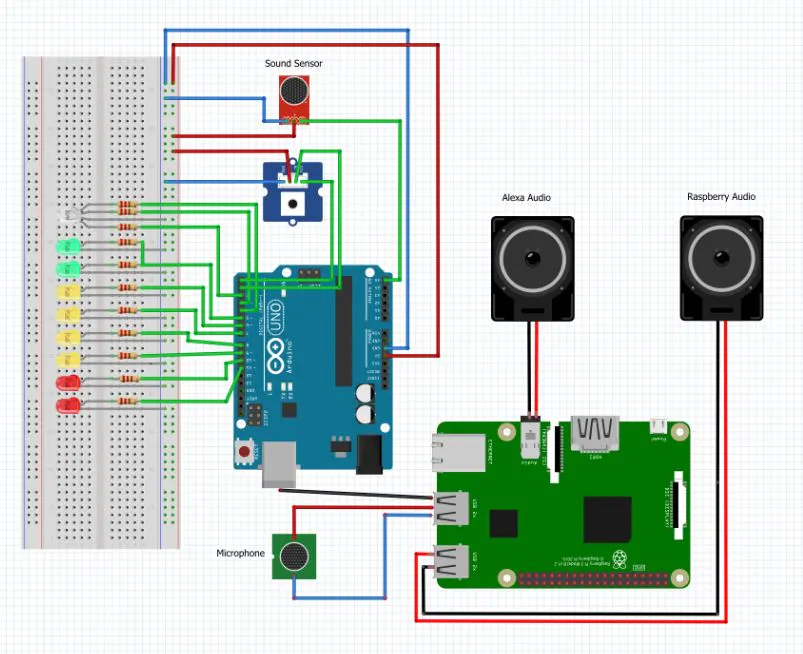### High-Level Schema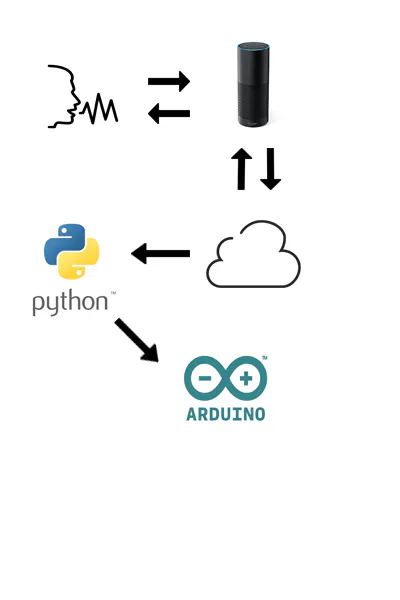## Code

### Server.py

Python
Python script
```from flask import Flask
from mutagen.mp3 import MP3

import serial
import time
import vlc

player = vlc.MediaPlayer()
#counter needed to know if we are at the first or the second question
counter = 0

#data strcuture that stores the informations that will be used to calculate the resultant mood
state = {
"Happy": 0,
"Neutral": 0,
"Angry": 0,
}

#create the serial channel for the communication wih Arduino
Arduino = serial.Serial("/dev/ttyACM0",9600)

def calculateState( mood ):
global counter
global state

counter += 1
if counter == 1:
state[mood]= 1
elif counter == 2:
counter = 0
state[mood]= 2
return statement("Trying to calculate your state...")

#here we intercept the event fired from Alexa when someone trigger our custom skill
def handler():
#we use global variable in order to change values for every events and functions
global counter
global player
#here we check if another song is currently playing
if player.is_playing():
return statement("Humour dj is already playing a song, wait!")
#if not, we send the "Welcome message" and we ask the first question
else:
return question("Hi! Welcome to the Alexa Humour DJ ! I can play a song for you based on your current mood. First of all, how are you?")

#here we handle the classified response for the four moods
def happy():
global counter
global state
counter += 1
#if we have asked only the first question, counter==1 and we ask the second
if counter == 1:
state["Happy"]+= 1
return question("And now, tell me, how was your day?")
#else we go and calculate the mood with the chooseSong() function
elif counter == 2:
counter = 0
state["Happy"]+= 1
#decisione della canzone in base allo stato d'animo
chooseSong()

return statement("This is the song  i chose for you, i hope you like it!")

#same as above, for every mood
def neutral():
global counter
global state
counter += 1
if counter == 1:
state["Neutral"]+= 1
return question("And now, tell me, how was your day?")
elif counter == 2:
counter = 0
state["Neutral"]+= 1

chooseSong()

return statement("This is the song  i chose for you, i hope you like it!")

def angry():
global counter
global state
counter += 1
if counter == 1:
state["Angry"]+= 1
return question("And now, tell me, how was your day?")
elif counter == 2:
counter = 0
state["Angry"]+= 1
chooseSong()

return statement("This is the song  i chose for you, i hope you like it!")

global counter
global state
global player

counter += 1
if counter == 1:
return question("And now, tell me, how was your day?")
elif counter == 2:
counter = 0
chooseSong()
return statement("This is the song  i chose for you, i hope you like it!")

#here is the function that we use to calculate the mood, launch the related song and send a signal to Ardunino
def chooseSong():
global ser
global player
#if Happy
if state["Happy"] == 2 or ( state["Happy"] ==1 and state["Angry"]==1  ) or (state["Happy"] ==1 and state["Sad"]==1 ):
player = vlc.MediaPlayer("/home/pi/Desktop/Happy/PharrellWilliams-Happy.mp3")
player.play()
Arduino.write(b'H')

#if Neutral
if state["Neutral"] == 2 or (state["Happy"] ==1 and state["Neutral"]==1  ):
player = vlc.MediaPlayer("/home/pi/Desktop/Neutral/Riders_on_the_Storm.mp3")
player.play()
Arduino.write(b'N')

#if Angry
if state["Angry"] == 2 or (state["Angry"]==1 and state["Sad"]==1  ) or (state["Angry"]==1 and state["Neutral"]==1 ):
player = vlc.MediaPlayer("/home/pi/Desktop/Angry/Enter_Sandman.mp3")
player.play()
Arduino.write(b'A')

player.play()
Arduino.write(b'S')
state = {"Happy": 0, "Neutral": 0,"Angry": 0, "Sad" : 0}

return;

#the server listens on localhost:5000
if __name__ == '__main__':
app.run(host='localhost', port = 5000, debug = True)
```

### ArduinoSketch

Arduino
```#include <ChainableLED.h>
ChainableLED leds1(2,3,1);
// Led and sensors
int pin_A0 = A5;
int pin_led_A = 5;
int pin_led_B = 6;
int pin_led_C = 7;
int pin_led_D = 8;
int pin_led_E = 9;
int pin_led_F = 10;
int pin_led_G = 11;
int pin_TouchPin=4;

//Variables
char mood=0;
int TouchValue = 0;
int valore_A0 = 0;
int on = 1;

void setup()
{
Serial.begin(9600);
//Inputs
pinMode(pin_A0, INPUT);
pinMode(pin_TouchPin, INPUT);
//Outputs
pinMode(pin_led_A, OUTPUT);
pinMode(pin_led_B, OUTPUT);
pinMode(pin_led_C, OUTPUT);
pinMode(pin_led_D, OUTPUT);
pinMode(pin_led_E, OUTPUT);
pinMode(pin_led_F, OUTPUT);
pinMode(pin_led_G, OUTPUT);
leds1.init();

}
void loop() {
// put your main code here, to run repeatedly:

if ( on == 1 ) {
}

//Low sounds
if(valore_A0 <= 168){
digitalWrite(pin_led_A, LOW);
digitalWrite(pin_led_B, LOW);
digitalWrite(pin_led_C, LOW);
digitalWrite(pin_led_D, LOW);
digitalWrite(pin_led_E, LOW);
digitalWrite(pin_led_F, LOW);
digitalWrite(pin_led_G, LOW);

}
if (valore_A0 > 168 && valore_A0 < 171)
{
digitalWrite(pin_led_A, HIGH);
digitalWrite(pin_led_B, LOW);
digitalWrite(pin_led_C, LOW);
digitalWrite(pin_led_D, LOW);
digitalWrite(pin_led_E, LOW);
digitalWrite(pin_led_F, LOW);
digitalWrite(pin_led_G, LOW);
}
//Middle sounds
if (valore_A0 >= 171 && valore_A0 < 174)
{
digitalWrite(pin_led_A, HIGH);
digitalWrite(pin_led_B, HIGH);
digitalWrite(pin_led_C, LOW);
digitalWrite(pin_led_D, LOW);
digitalWrite(pin_led_E, LOW);
digitalWrite(pin_led_F, LOW);
digitalWrite(pin_led_G, LOW);
}

if (valore_A0 >= 174 && valore_A0 <176)
{
digitalWrite(pin_led_A, HIGH);
digitalWrite(pin_led_B, HIGH);
digitalWrite(pin_led_C, HIGH);
digitalWrite(pin_led_D, LOW);
digitalWrite(pin_led_E, LOW);
digitalWrite(pin_led_F, LOW);
digitalWrite(pin_led_G, LOW);
}
if (valore_A0 >= 176 && valore_A0 < 180)
{
digitalWrite(pin_led_A, HIGH);
digitalWrite(pin_led_B, HIGH);
digitalWrite(pin_led_C, HIGH);
digitalWrite(pin_led_D, HIGH);
digitalWrite(pin_led_E, LOW);
digitalWrite(pin_led_F, LOW);
digitalWrite(pin_led_G, LOW);
}
if (valore_A0 >= 180 && valore_A0 < 190)
{
digitalWrite(pin_led_A, HIGH);
digitalWrite(pin_led_B, HIGH);
digitalWrite(pin_led_C, HIGH);
digitalWrite(pin_led_D, HIGH);
digitalWrite(pin_led_E, HIGH);
digitalWrite(pin_led_F, LOW);
digitalWrite(pin_led_G, LOW);
}
//High sounds
if (valore_A0 >= 190 && valore_A0 < 220)
{
digitalWrite(pin_led_A, HIGH);
digitalWrite(pin_led_B, HIGH);
digitalWrite(pin_led_C, HIGH);
digitalWrite(pin_led_D, HIGH);
digitalWrite(pin_led_E, HIGH);
digitalWrite(pin_led_F, HIGH);
digitalWrite(pin_led_G, LOW);
}

if (valore_A0 >= 270)
{
digitalWrite(pin_led_A, HIGH);
digitalWrite(pin_led_B, HIGH);
digitalWrite(pin_led_C, HIGH);
digitalWrite(pin_led_D, HIGH);
digitalWrite(pin_led_E, HIGH);
digitalWrite(pin_led_F, HIGH);
digitalWrite(pin_led_G, HIGH);
}
delay(50);

switch (mood){
case 'A': //angry
{
leds1.setColorRGB(0,255,0,0);
break;
}

{
leds1.setColorRGB(0,0,0,255);
break;
}

case 'N': //neutral
{
leds1.setColorRGB(0,255,255,255);
break;
}

case 'H': //happiness
{
leds1.setColorRGB(0,0,255,0);
break;
}

default:
Serial.flush();
}

//"Turn off" Arduino
if (TouchValue == 1)
{
leds1.setColorRGB(0,0,0,0);
mood='Z';
valore_A0 = 0;
on = 0;
digitalWrite(pin_led_A, LOW);
digitalWrite(pin_led_B, LOW);
digitalWrite(pin_led_C, LOW);
digitalWrite(pin_led_D, LOW);
digitalWrite(pin_led_E, LOW);
digitalWrite(pin_led_F, LOW);
digitalWrite(pin_led_G, LOW);

}

}
```

## Credits

### Frao92

1 project • 5 followers

### Filippo Leoncini

0 projects • 4 followers

### Valeria

0 projects • 4 followers

### David

0 projects • 2 followers

### Erik degiorgis

0 projects • 2 followers